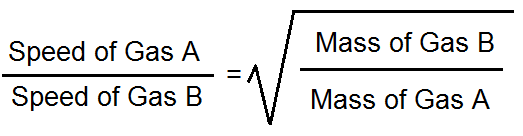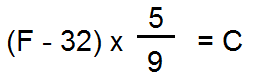# States of Matter Flashcards

States of Matter Flashcards
1/18 (missed) 0 0
Create Your Account To Continue Studying

As a member, you'll also get unlimited access to over 75,000 lessons in math, English, science, history, and more. Plus, get practice tests, quizzes, and personalized coaching to help you succeed.

Try it risk-free for 30 days. Cancel anytime.
###### Already registered? Log in here for access
Define the term: Effusion
Molecules of gas leak out of a container
Got it
Graham's Law gives this formula for comparing the effusion speed of two gases.
Speed A / Speed B = sqrt(Mass B / Mass A)Got it
Boyle's Law gives this formula for the effect of changes in pressure or volume on gases in a closed container at constant temperature.
P 1 * V 1 = P 2 * V 2 , where P 1 & P 2 stand for pressure, before & after a change, respectively. V 1 & V 2 stand for volume, before & after a change, respectively.
Got it
The relative size of one degree-unit in Celsius vs. one degree-unit Fahrenheit
Celsius units are larger than Fahrenheit units. (The span from freezing to boiling is 180 units of Fahrenheit degrees, but it's only 100 units of Celsius degrees.)
Got it
The relative size of one degree-unit Celsius vs. one Kelvin
They're exactly the same.
Got it
How to convert degrees Fahrenheit to degrees Celsius
(Fahrenheit minus 32) times five ninthsGot it
How to convert from degrees Celsius to Kelvins
Add 273 to the temperature in Celsius.
Got it
How pressure and volume are related in an ideal gas
When pressure goes up, volume goes down. When pressure goes down, volume goes up.
Got it
In an ideal gas, how average kinetic energy of the molecules relates to temperature
Average kinetic energy is directly proportional to temperature
Got it

### Ready to move on?

or choose a specific lesson: See all lessons in this chapter
18 cards in set

## Flashcard Content Overview

In this flashcard set, you can learn properties and principles of how the states of matter - solid, liquid, and gas - interact. The flashcards cover many aspects of the ideal gas law, including ways in which real gases deviate from ideal behaviors. You will also find cards that cover other gas laws, including Graham's Law about effusion and Boyle's Law about changes in pressure and volume.

You'll test your knowledge of the formulas for converting degrees Fahrenheit to degrees Celsius and converting degrees Celsius to Kelvins. Other flashcards help you refresh your memory about heating curves and phase diagrams, including key terminology like heat of vaporization, sublimation, and the critical point.

Front
Back
In an ideal gas, how average kinetic energy of the molecules relates to temperature
Average kinetic energy is directly proportional to temperature
How pressure and volume are related in an ideal gas
When pressure goes up, volume goes down. When pressure goes down, volume goes up.
How to convert from degrees Celsius to Kelvins
Add 273 to the temperature in Celsius.
How to convert degrees Fahrenheit to degrees Celsius
(Fahrenheit minus 32) times five ninthsThe relative size of one degree-unit Celsius vs. one Kelvin
They're exactly the same.
The relative size of one degree-unit in Celsius vs. one degree-unit Fahrenheit
Celsius units are larger than Fahrenheit units. (The span from freezing to boiling is 180 units of Fahrenheit degrees, but it's only 100 units of Celsius degrees.)
Boyle's Law gives this formula for the effect of changes in pressure or volume on gases in a closed container at constant temperature.
P 1 * V 1 = P 2 * V 2 , where P 1 & P 2 stand for pressure, before & after a change, respectively. V 1 & V 2 stand for volume, before & after a change, respectively.
Graham's Law gives this formula for comparing the effusion speed of two gases.
Speed A / Speed B = sqrt(Mass B / Mass A)Define the term: Effusion
Molecules of gas leak out of a container
In the ideal gas law, R stands for this.
0.0821 (atm * L) / (mol * K)
How molecule mass changes the Maxwell-Boltzmann distribution
Heavier molecules move more slowly than lighter ones, so the curve is higher at the low end, and less broad.
Define the term: Heat of vaporization
The amount of heat that a substance must have absorbed in order to completely change from liquid to steam.
The two main assumptions scientists make about ideal gases

(1) The gas particles themselves have negligible volume.

(2) The intermolecular attraction between gas molecules is negligible.

Factors that make a gas behave LESS like an ideal gas
Intermolecular forces between particles of the gas, such as dipole-dipole interactions; mass of the gas molecules
The plateau on a heating curve represents this.
The substance is starting to melt or vaporize. Only at the end of the plateau is the transition complete.
Define the term: Sublimation
A solid material becoming a gas without being a liquid in between
Define the term: Critical point (on a phase diagram)
Above this point, the gas and liquid phases of a substance blend into one phase called a supercritical fluid.
Define the term: Supercritical fluid
The blended gas + liquid forms of a substance, which can't be separated at certain temperature + pressure conditions

To unlock this flashcard set you must be a Study.com Member.
Create your account

### Unlock Your Education

#### See for yourself why 30 million people use Study.com

##### Become a Study.com member and start learning now.

Already a member? Log In

Support